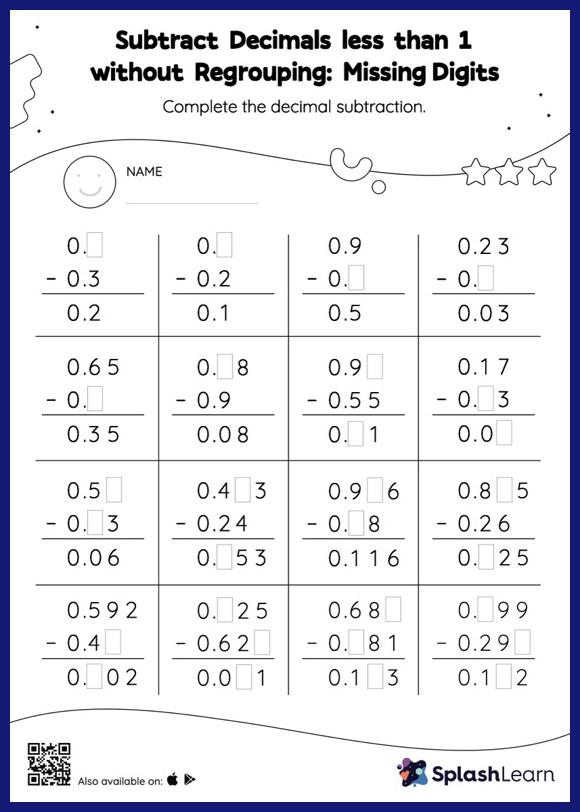# Subtract Decimals less than 1 without Regrouping: Missing Digits Worksheet

Home > Subtract Decimals less than 1 without Regrouping: Missing DigitsChallenge your young math wizards to solve this subtract decimals less than 1 without regrouping worksheet. While subtracting decimals, students use the relationship between addition and subtraction to find the missing number. They do not need to regroup numbers in subtract decimals less than 1 without regrouping worksheet.